# 泛型编程

2019/04/10 10:10

## 目录

1. 函数模板

1.1 函数模板的来源

1.2 函数模板的定义

1.3 函数模板的特点

1.4 函数模板的语法规则

1.5 函数模板的调用方式

1.6 多参数的函数模板

1.7 函数模板与函数重载

1.8 函数模板的实现机制（两次编译）

2. 类模板

2.1 类模板的概念和意义

2.2 类模板的语法规则

2.3 类模板的实现机制（两次编译）

2.4 类模板在工程应用中的推荐写法

2.5 类模板的特化（包括函数模板的特化）

2.6 关于特化的一些问题

顾名思义泛型编程就是使用“泛型”的思想去写代码，这里的“泛型”指的是将数据类型作为参数传递（类型参数化）；换言之 泛型编程 是 一种不考虑具体数据类型的编程方式，其典型代表就是STL（Standard Template Library 标准模板库）。

1. 如果将泛型编程的思想应用于函数中，就产生了函数模板（通用函数）；

2. 同理，将泛型编程的思想应用于类中，就会产生类模板（通用类）；

接下来就分别介绍这两种技术：函数模板、类模板。

## 函数模板（Function Template）

### 1、函数模板的来源

为了更加深刻的理解函数模板，我们可以用一个例子说明情况。比如，现在要交换两个变量的值，怎么做？就目前来看有2种方法，分别是 使用宏代码块 和 使用函数定义（函数重载）；1 #include <iostream>
2 #include <string>
3
4 using namespace std;
5
6 #define SWAP(t, a, b)    \
7 do                       \
8 {                        \
9     t c = a;             \
10     a = b;               \
11     b = c;               \
12 }while(0)
13
14 int main()
15 {
16     int a = 10;
17     int b = 11;
18
19     SWAP(int, a, b);
20
21     cout << "a = " << a << endl;
22     cout << "b = " << b << endl;
23
24     double m = 12;
25     double n = 13;
26
27     SWAP(double, m, n);
28
29     cout << "m = " << m << endl;
30     cout << "n = " << n << endl;
31
32     string s1 = "c++";
33     string s2 = "python";
34
35     SWAP(string, s1, s2);
36
37     cout << "s1 = " << s1 << endl;
38     cout << "s2 = " << s2 << endl;
39
40     return 0;
41
42 }
43 /**
44 * a = 11
45 * b = 10
46 * m = 13
47 * n = 12
48 * s1 = python
49 * s2 = c++
50 */1 #include <iostream>
2 #include <string>
3
4 using namespace std;
5
6 void Swap(int& a, int& b)
7 {
8     int c = a;
9     a = b;
10     b = c;
11 }
12
13 void Swap(double& a, double& b)
14 {
15     double c = a;
16     a = b;
17     b = c;
18 }
19
20 void Swap(string& a, string& b)
21 {
22     string c = a;
23     a = b;
24     b = c;
25 }
26
27
28 int main()
29 {
30     int a = 10;
31     int b = 11;
32
33     Swap(a, b);
34
35     cout << "a = " << a << endl;
36     cout << "b = " << b << endl;
37
38     double m = 12;
39     double n = 13;
40
41     Swap(m, n);
42
43     cout << "m = " << m << endl;
44     cout << "n = " << n << endl;
45
46     string s1 = "c++";
47     string s2 = "python";
48
49     Swap(s1, s2);
50
51     cout << "s1 = " << s1 << endl;
52     cout << "s2 = " << s2 << endl;
53
54     return 0;
55
56 }
57 /**
58 * a = 11
59 * b = 10
60 * m = 13
61 * n = 12
62 * s1 = python
63 * s2 = c++
64 */

通过案列可知，这2种方法都可以实现功能，但是它们各自都有缺点。

定义宏代码块

优点：代码复用，适合所有的类型；

缺点：缺少类型检查；

定义函数

优点：真正的函数调用，有类型检查；

缺点：根据类型重复定义函数，无法代码复用；

那么，有没有一种方法可以同时拥有上述2种方法的优点（1. 代码复用，适合所有类型； 2. 类型检查）呢？--- 当然有了，那就是 函数模板

### 2、函数模板的定义

所谓函数模板，实际上是建立一个通用函数，它所用到的数据类型（包括返回值类型、形参类型、局部变量类型）可以不具体指定，而是用一个虚拟的类型来代替（实际上是用一个标识符来占位）；当发生函数调用时再根据传入的实参来自动推导出真正的数据类型。

在函数模板中，数据的值和类型都被参数化了，发生函数调用时编译器会根据传入的实参来推演形参的值和类型。换个角度说，函数模板除了支持值的参数化，还支持类型的参数化

只要定义了函数模板，就可以将类型参数用于函数定义和函数声明了。

### 3、函数模板的特点

1. 在函数定义时可以不指明具体的数据类型；--- 函数定义

2. 一种特殊的函数，可用不同的类型进行调用；---  函数调用

3. 看起来与普通函数很相似，区别是类型被参数化（当发生函数调用时，数据的类型可以通过参数来传递，编译器根据传入的实参自动推断数据类型。--- 参数传递

### 4、函数模板的语法规则

template <typename T>

template <typename T, typename T1, typename T2, ...>　　多参数的函数模板， T 第一个类型参数，T1 是第二个类型参数...

template 关键字用于声明开始进行泛型编程

typename 关键字用于声明泛指类型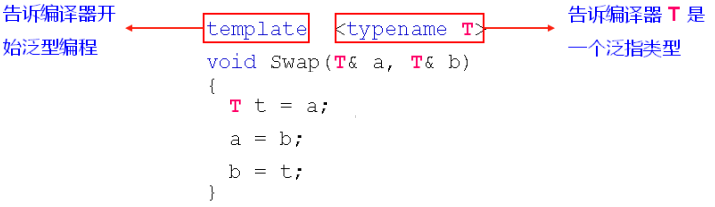1 #include <iostream>
2 #include <string>
3
4 using namespace std;
5
6 template < typename T >
7 void Swap(T& a, T& b)
8 {
9     T c = a;
10     a = b;
11     b = c;
12 }
13
14 template < typename T >
15 void Sort(T a[], int len)
16 {
17     for(int i=0; i<len-1; i++)
18     {
19         for(int j=i+1; j<len; j++)
20         {
21             if( a[i] > a[j] )
22             {
23                 Swap(a[i], a[j]);
24             }
25         }
26     }
27 }
28
29 template < typename T >
30 void Print(T a[], int len)
31 {
32     for(int i=0; i<len; i++)
33     {
34         cout << a[i] << ", ";
35     }
36
37     cout << endl;
38 }
39
40 int main()
41 {
42     int a = {5, 3, 2, 4, 1};
43
44     cout << "排序前：";
45     Print(a, 5);
46     Sort(a, 5);
47     cout << "排序后：";
48     Print(a, 5);
49
50     string s = {"Java", "C++", "Python", "Html", "Matlab"};
51
52     cout << "排序前：";
53     Print(s, 5);
54     Sort(s, 5);
55     cout << "排序后：";
56     Print(s, 5);
57
58     return 0;
59 }
60 /**
61  * 排序前：5, 3, 2, 4, 1,
62  * 排序后：1, 2, 3, 4, 5,
63  * 排序前：Java, C++, Python, Html, Matlab,
64  * 排序后：C++, Html, Java, Matlab, Python,
65 */

5、函数模板的调用方式

！！！发生函数调用时，函数模板会根据 实参   对  参数类型   进行推导，但无法自动推导返回值类型；

！！！函数模板本身不允许隐式类型转换

1. 自动推导调用方式（实参类型与泛指类型必须严格匹配，不能进行隐式类型转换）；

2. 具体类型显示调用（显示的指定数据类型，可以进行隐式类型转换）

3. 自动推导 + 显示调用 二者结合（在多参数的函数模板中使用）；

 1 template <typename T>
2 void Swap(T& a, T& b)
3 {
4       T c = a;
5       a = b;
6       b = c;
7 }
8
9 // 测试 1
10 int a = 1；
11 int b = 2;
12 Swap(a, b);         // 自动推导调用方式
13 Swap<int>(a, b);    // 具体类型显示调用
14
15 // 测试 2
16 int a = 3；
17 float b = 4.5;
18 Swap(a, b);        // error，a, b 数据类型不一致，若此时进行函数调用，会产生二义性（如 void Swap(int a, int b); void Swap(float a, float b); ）
19 Swap<float>(a, b); // 若指定了数据类型为 float，即 T = float，在函数调用过程中（void Swap(float a, float b); ），a 进行了隐式类型转换

### 6、多参数的函数模板

可以从左向右部分指定类型参数，工程中将返回值类型作为第一个类型参数（这样方便进行自动推导）；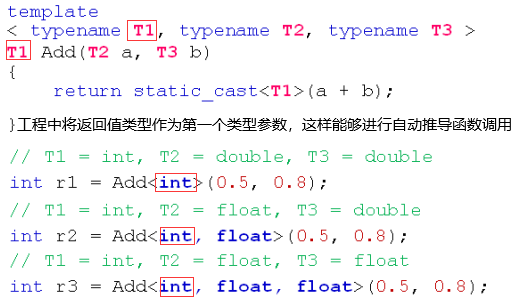1 #include <iostream>
2 #include <string>
3
4 using namespace std;
5
6 template
7 < typename T1, typename T2, typename T3 >
8 T1 Add(T2 a, T3 b)
9 {
10     return static_cast<T1>(a + b);
11 }
12
13 int main()
14 {
15     // T1 = int, T2 = double, T3 = double
16     int r1 = Add<int>(0.5, 0.8);
17
18     // T1 = double, T2 = float, T3 = double
19     double r2 = Add<double, float>(0.5, 0.8);
20
21     // T1 = float, T2 = float, T3 = float
22     float r3 = Add<float, float, float>(0.5, 0.8);
23
24     cout << "r1 = " << r1 << endl;     // r1 = 1
25     cout << "r2 = " << r2 << endl;     // r2 = 1.3
26     cout << "r3 = " << r3 << endl;     // r3 = 1.3
27
28     return 0;
29 }

### 7、函数模板与函数重载

调用规则：

1. 函数模板可以像普通函数一样被重载；

2. C++编译器优先考虑普通函数；

3. 如果函数模板可以产生一个更好的匹配，那么选择模板；

4. 可以通过 空模板实参列表 的语法限定编译器只通过模板匹配 。// func<>(a,b)；

！！！函数模板本身不允许隐式类型转换；

！！！函数模板支持完全特化（在类模板中介绍）

！！！在重载函数模板时，优先选择函数模板特化，其次才是函数重载。1 #include <iostream>
2 #include <string>
3
4 using namespace std;
5
6
7 template < typename T >
8 T Max(T a, T b)
9 {
10     cout << "T Max(T a, T b)" << endl;
11
12     return a > b ? a : b;
13 }
14
15 int Max(int a, int b)
16 {
17     cout << "int Max(int a, int b)" << endl;
18
19     return a > b ? a : b;
20 }
21
22 template < typename T >
23 T Max(T a, T b, T c)
24 {
25     cout << "T Max(T a, T b, T c)" << endl;
26
27     return Max(Max(a, b), c);
28 }
29
30 int main()
31 {
32     cout << Max(1, 2) << endl;          // 普通函数 Max(int, int)
33     cout << Max<>(1, 2) << endl;        // 函数模板 Max<int>(int, int)
34     cout << Max(3.0, 4) << endl;        // 普通函数 Max(int, int)
35     cout << Max(3.0, 4.0) << endl;      // 函数模板 Max<double>(double, double)
36     cout << Max(5.0, 6.0, 7.0) << endl; // 函数模板 Max<double>(double, double, double)
37
38     return 0;
39 }
40 /**
41  * int Max(int a, int b)
42  * 2
43  * T Max(T a, T b)
44  * 2
45  * int Max(int a, int b)
46  * 4
47  * T Max(T a, T b)
48  * 4
49  * T Max(T a, T b, T c)
50  * T Max(T a, T b)
51  * T Max(T a, T b)
52  * 7
53 */

### 8、函数模板的实现机制

1. 编译器从函数模板通过具体类型产生不同的函数；

2. 编译器会对函数模板进行两次编译

（1）对模板代码本身进行编译；

（2）对参数替换后的代码进行编译；1 #include <iostream>
2 #include <string>
3
4 using namespace std;
5
6 class Test
7 {
8     //Test(const Test&);
9 public:
10     Test()
11     {
12     }
13 };
14
15 template < typename T >
16 void Swap(T& a, T& b)
17 {
18     T c = a;  // 当T=Test时，第二次编译就会调用拷贝构造函数（private），此时编译失败
19     a = b;
20     b = c;
21 }
22
23 typedef void(FuncI)(int&, int&);
24 typedef void(FuncD)(double&, double&);
25 typedef void(FuncT)(Test&, Test&);
26
27 int main()
28 {
29     FuncI* pi = Swap;    // 编译器自动推导 T 为 int
30     FuncD* pd = Swap;    // 编译器自动推导 T 为 double
31     FuncT* pt = Swap;    // 编译器自动推导 T 为 Test
32
33     cout << "pi = " << reinterpret_cast<void*>(pi) << endl; // pi = 0x400a0a
34     cout << "pd = " << reinterpret_cast<void*>(pd) << endl; // pd = 0x400a37
35     cout << "pt = " << reinterpret_cast<void*>(pt) << endl; // pt = 0x400a70
36
37     return 0;
38 }

## 类模板（Class Template）

### 1、 类模板的概念和意义

在C++ 中，将模板（泛型）的思想应用于类，使得类的实现不在关注数据元素的具体类型，而只关注类所需要实现的功能，这就是c++中的的类模板。总之，类模板就是以相同的方式去处理不同类型的数据。

在STL中有很多类（数组类、链表类、Stack 类、Queue 类等），这些类主要用于存储和组织数据元素，且这些类的实现与具体的数据类型无关；这样做的好处是统一的算法应用在不同的数据类型之间。那么，在STL中，这项技术是如何实现的？--- 通过 类模板 实现。

由此可见，类模板非常适合编写数据结构的相关代码。

### 2、类模板的语法规则

template <typename T>

template <typename T1, typename T2, typename T3, ...>　　多参数的类模板声明， T1 第一个类型参数，T2 是第二个类型参数...

template 关键字用于声明开始进行泛型编程

typename 关键字用于声明泛指类型

声明的泛指类型 T 可以出现在类模板的任意地方；（以上内容与函数模板一样）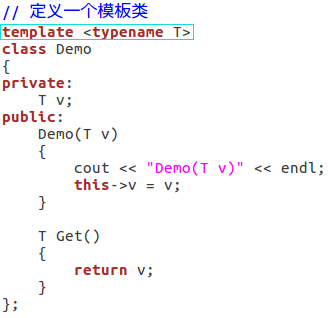！！！在类模板创建对象时，必须显示指定具体类型，不能自动推导；可以通过使用具体类型<Type>创建对象1 #include <iostream>
2
3 using namespace std;
4
5 // 定义一个模板类
6 template <typename T>
7 class Demo
8 {
9 private:
10     T v;
11 public:
12     Demo(T v)
13     {
14         cout << "Demo(T v)" << endl;
15         this->v = v;
16     }
17
18     T Get()
19     {
20         return v;
21     }
22 };
23
24 int main()
25 {
26     // 使用类模板创建对象时，必须显示指定类型！！！
27     Demo<int> d1(10);
28     cout << d1.Get() << endl;
29
30     Demo<string> d2("template test");
31     cout << d2.Get() << endl;
32
33     return 0;
34 }
35 /**
36  * Demo(T v)
37  * 10
38  * Demo(T v)
39  * template test
40 */

### 3、 类模板的实现机制（两次编译）

编译器对类模板的处理方式和函数模板相同，即使用相同的方式处理不同的类型，都会经过两次编译。

1. 在声明的地方对类模板代码本身进行编译；

2. 在使用的地方对参数替换后的代码进行编译；（！！！部分编译，仅仅对所调用的代码进行编译

怎么证明类模板是使用两次编译的？

为了说明问题，可以使用字符串测试。当 两个字符串直接相加时，其实是对这两个字符串进行拼接，如”string“ + ”666“ = ”string666“；但是，当字符串与数字相加时，程序在编译时就会报错，因为在string类中没有重载这种操作。

测试流程：

首先，定义一个类模板，接下来用类模板分别创建对象；

 1 template < typename T1, typename T2 >
2 class Demo
3 {
4 public:
5     Demo()
6     {
7         cout << "Demo()" << endl;
8     }
9
10     T1 joint(T1 a, T2 b)
11     {
12         cout << "T1 joint(T1 a, T2 b)" << endl;
13         return a + b;
14     }
15 };

若使用如下测试语句，程序正常执行：

 1 // 第二次编译，编译对象d1对应的构造函数，此时，T1=String，T2=String
2 Demo<string, string> d1;
3 // 第二次编译，编译对象d1对应的成员函数 T1 joint(T1 a, T2 b){..}
4 cout << d1.joint("Test", "_string") << endl;
5
6 /**
7  * 运行结果
8  * Demo()
9  * T1 joint(T1 a, T2 b)
10  * Test_string
11 */

若使用如下测试语句，程序则报错：

 1 // 第二次编译，编译对象d2对应的构造函数，此时，T1=String，T2=int
2 Demo<string, int> d2;
3 /*
4 第二次编译，编译对象d2对应的成员函数
5    T1 joint(T1 a, T2 b)
6     {
7         cout << "T1 joint(T1 a, T2 b)" << endl;
8         return a + b;  // ！！！报错
9     }
10 */
11 cout << d2.joint("Test", 3) << endl;

报错原因：String 与 int 不能直接相加，需要重载+操作符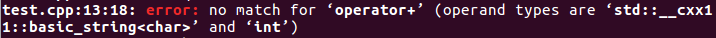修改错误，添加如下代码：

 1 // 如 ”abc“ + 3 = ”abcabcabc“
2 string operator+(string &l, int r)
3 {
4     string ret = "";
5
6     while(r)
7     {
8         ret += l;
9         r--;
10     }
11
12     return ret;
13 }

程序可以正常运行，运行结果为：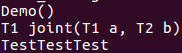1 #include <iostream>
2 #include <string>
3
4 using namespace std;
5
6 template < typename T1, typename T2 >
7 class Demo
8 {
9 public:
10     Demo()
11     {
12         cout << "Demo()" << endl;
13     }
14
15     T1 joint(T1 a, T2 b)
16     {
17         cout << "T1 joint(T1 a, T2 b)" << endl;
18         return a + b;
19     }
20 };
21
22 string operator+(string &l, int r)
23 {
24     string ret = "";
25
26     while(r)
27     {
28         ret += l;
29         r--;
30     }
31
32     return ret;
33 }
34
35
36 int main()
37 {
38     Demo<string, string> d1;
39
40     cout << d1.joint("Test", "_string") << endl;
41
42     Demo<string, int> d2;
43
44     cout << d2.joint("Test", 3) << endl;
45
46     return 0;
47 }
48
49 /**
50  * 运行结果：
51  * Demo()
52  * joint(T1 a, T2 b)
53  * Test_string
54  * Demo()
55  * joint(T1 a, T2 b)
56  * TestTestTest
57  */

### 4、类模板在工程应用中的推荐写法

1. 类模板必须在头文件中定义(.hpp文件)；

2. 类模板不能分开实现在不同的文件中（声明与定义必须在同一个文件中）；

3. 类模板外部定义的成员函数需要加上模板 <> 声明；

注：以上三条规则不是 C++ 和编译器的一部分，只是工程应用里习惯这样做；这样做后，代码可维护性、扩展性都会变好，因此建议遵守这三条规则；1 // 头文件 test.hpp
2 #ifndef _TEST_H
3 #define _TEST_H
4
5 #include <iostream>
6 #include <string>
7
8 using namespace std;
9
10 template < typename T1, typename T2 >
11 class Demo
12 {
13 public:
14     Demo();
15     T1 joint(T1 a, T2 b);
16 };
17
18 template < typename T1, typename T2 >
19 Demo<T1, T2>::Demo()
20 {
21     cout << "Demo()" << endl;
22 }
23
24 template < typename T1, typename T2 >
25 T1 Demo<T1, T2>::joint(T1 a, T2 b)
26 {
27     cout << "T1 joint(T1 a, T2 b)" << endl;
28     return a + b;
29 }
30
31 // 全局函数
32 string operator+(string &l, int r)
33 {
34     string ret = "";
35
36     while(r)
37     {
38         ret += l;
39         r--;
40     }
41
42     return ret;
43 }
44
45 #endif
46
47 // main.cpp
48
49 #include <iostream>
50 #include <string>
51 #include "test.hpp"
52
53 using namespace std;
54
55 int main()
56 {
57     Demo<string, string> d1;
58
59     cout << d1.joint("Test", "_string") << endl;
60
61     Demo<string, int> d2;
62
63     cout << d2.joint("Test", 3) << endl;
64
65     return 0;
66 }
67
68 /**
69  * 运行结果：
70  * Demo()
71  * joint(T1 a, T2 b)
72  * Test_string
73  * Demo()
74  * joint(T1 a, T2 b)
75  * TestTestTest
76  */

### 5、类模板的特化

“特化”  由两部分组成：

1. 部分特化：任何针对模版参数进一步进行条件限制设计的特化版本；（模板条件<>中必须有 泛指类型T，可以与其它类型一起使用）

2. 完全特化：针对所有的模版参数进行特化；（模板条件<>中都是具体类型，不包括 泛指类型T）

类模板“特化”的注意事项：

1. “特化” 就是指定类模板的特定实现

2. “特化” 是根据类型参数分开实现类模板，本质还是同一个类模板

3. “特化”类模板的使用方式是统一的，必须显示的指定每一个类型参数

类模板的特化：

 1 // 类模板
2 template<typename T1, typename T2>
3 class Demo{};
4
5 // 部分特化
6 template<typename T, typename int>
7 class Demo<T, int>{};
8
9 template<typename T>
10 class Demo<T, T>{};
11
12 template<typename T1, typename T2>
13 class Demo<T1*, T2*>{};
14
15 // 完全特化
16 template<>
17 class Demo<int, int>{};
18
19 template<>
20 class Demo<const char*, int>{};

补充：函数模板的特化（只支持完全特化）

// 函数模板
template <typename T>
void Equal(T a, T b){};

// 函数模板的完全特化
template <>
void Equal<double>(double a, double b){};

// ！！！函数模板不支持部分特化1 #include <iostream>
2 #include <string>
3
4 using namespace std;
5
6 template
7 < typename T1, typename T2 >
8 class Demo
9 {
10 public:
11     void joint(T1 a, T2 b)
12     {
13         cout << "类模板：void joint(T1 a, T2 b)" << endl;
14         cout << a << b << endl;
15     }
16 };
17
18 template
19 <typename T1, typename T2 >
20 class Demo<T1*, T2*>    // 关于指针的特化实现
21 {
22 public:
23     void joint(T1* a, T2* b)
24     {
25         cout << "部分指针特化：void joint(T1* a, T2* b)" << endl;
26         cout << *a << *b << endl;
27     }
28 };
29
30 template
31 <typename T>
32 class Demo<T, T>    // 当 Demo 类模板的两个类型参数完全相同时，使用这个实现
33 {
34 public:
35     void joint(T a, T b)
36     {
37         cout << "部分特化：void joint(T a, T b)" << endl;
38         cout << a << b << endl;
39     }
40 };
41
42 template
43 <>
44 class Demo<char*, char*>    // 当T1 = char*, T2 = char*,使用这个实现
45 {
46 public:
47     void joint(const char* a, const char* b)
48     {
49         cout << "完全指针特化：void joint(char* a, char* b)" << endl;
50         cout << a << b << endl;
51     }
52 };
53
54 template
55 <>
56 class Demo<string, int>     // 当T1 = string, T2 = int,使用这个实现
57 {
58 public:
59     void joint(string a, int b)
60     {
61         string ret = "";
62
63         while(b)
64         {
65             ret += a;
66             b--;
67         }
68
69         cout << "完全特化：void joint(string a, int b)" << endl;
70         cout << ret << endl;
71     }
72 };
73
74
75 int main()
76 {
77     Demo<string, string> d1;
78     d1.joint("Test", "_string");
79
80     Demo<int, int> d2;
81     d2.joint(12, 34);
82
83     Demo<char*, char*> d3;
84     d3.joint("Test", "_char*");
85
86     Demo<string, int> d4;
87     d4.joint("Test", 3);
88
89     Demo<int*, float*> dd;
90     int a = 13;
91     float b = 14;
92     dd.joint(&a, &b);
93
94     Demo<const char*, string> d;
95     d.joint("12", ".34");
96
97     return 0;
98 }
99
100 /**
101  * 运行结果：
102  * 部分特化：void joint(T a, T b)
103  * Test_string
104  * 部分特化：void joint(T a, T b)
105  * 1234
106  * 完全指针特化：void joint(char* a, char* b)
107  * Test_char*
108  * 完全特化：void joint(string a, int b)
109  * TestTestTest
110  * 部分指针特化：void joint(T1* a, T2* b)
111  * 1314
112  * 类模板：void joint(T1 a, T2 b)
113  * 12.34
114  * /

由上述案列可知，对象调用对应成员函数的优先顺序为：完全特化  > 部分特化 > 类模板

### 6、关于特化的一些问题

1. 类模板特化与重定义的差异：

重定义：

（1） 重定义必须实现两个类模板  或者是 一个类模板加上 一个新的类；

（2） 重定义之后，二者没有任何关系

（3） 使用的时候需要考虑如何选择的问题（因为重定义之后又多了一个类，所以类模板统一的使用方式被销毁）

特化：

（1） 特化只是模板的分开实现，本质还是同一个类模板

（2） 特化是类模板的一部分，而这一部分有自己专有的工作方式；

（3） 类模板特化后，能够以统一的方式使用类模板和特化类；（与类模板的使用方式相同）

结论：特化与重定义是实现同一功能的两种不同手段；在使用时，优先选择特化实现，只有特化解决不了才会重新考虑重定义。1 #include <iostream>
2 #include <string>
3
4 using namespace std;
5
6 template
7 < typename T1, typename T2 >
8 class Demo
9 {
10 public:
11     void joint(T1 a, T2 b)
12     {
13         cout << "类模板：void joint(T1 a, T2 b)" << endl;
14         cout << a << b << endl;
15     }
16 };
17
18 // 完全特化实现
19 template
20 <>
21 class Demo<string, int>     // 当T1 = string, T2 = int,使用这个实现
22 {
23 public:
24     void joint(string a, int b)
25     {
26         string ret = "";
27
28         while(b)
29         {
30             ret += a;
31             b--;
32         }
33
34         cout << "完全特化：void joint(string a, int b)" << endl;
35         cout << ret << endl;
36     }
37 };
38
39 // 重定义实现
40 class Demo_redefine
41 {
42 public:
43     void joint(string a, int b)
44     {
45         string ret = "";
46
47         while(b)
48         {
49             ret += a;
50             b--;
51         }
52
53         cout << "重定义：void joint(string a, int b)" << endl;
54         cout << ret << endl;
55     }
56 };
57
58 int main()
59 {
60     // 对于同一功能的不同实现方法：特化实现 与 重定义一个新类，优先选择 特化实现
61     // 特化实现
62     Demo<string, int> d4;
63     d4.joint("Test", 3);
64     // 重定义实现
65     Demo_redefine dr;
66     dr.joint("Test", 3);
67
68     return 0;
69 }
70
71 /**
72  * 运行结果：
73  * 完全特化：void joint(string a, int b)
74  * TestTestTest
75  * 重定义：void joint(string a, int b)
76  * TestTestTest
77  * /

2. 函数模板完全特化 与 函数重载

结论：

（1）函数模板特化 与 函数重载同时存在时，编译器优先选择函数重载，其次函数模板特化，最后，函数模板；

（2）工程建议：当需要重载函数模板时，优先考虑使用模板特化；当模板特化无法满足需求，再使用函数重载！1 #include <iostream>
2 #include <string>
3
4 using namespace std;
5
6 // 函数模板
7 template
8 < typename T >
9 bool Equal(T a, T b)
10 {
11     cout << "函数模板：bool Equal(T a, T b)" << endl;
12
13     return a == b;
14 }
15
16 // 函数模板完全特化
17 template
18 < >
19 bool Equal<double>(double a, double b)
20 {
21     const double delta = 0.00000000000001;
22     double r = a - b;
23
24     cout << "函数模板完全特化：bool Equal<double>(double a, double b)" << endl;
25
26     return (-delta < r) && (r < delta);
27 }
28
29 // 函数重载
30 bool Equal(double a, double b)
31 {
32     const double delta = 0.00000000000001;
33     double r = a - b;
34
35     cout << "函数重载：bool Equal(double a, double b)" << endl;
36
37     return (-delta < r) && (r < delta);
38 }
39
40 int main()
41 {
42     cout << Equal( 1, 1 ) << endl;          // Equal<int>(int, int)
43     cout << Equal( 1.1, 1.1 ) << endl;      // Equal(double, double) // 编译器优先选择普通函数
44     cout << Equal<>( 0.001, 0.001 ) << endl;// Equal<double>(double, double)
45
46     return 0;
47 }
48 // 注：不能直接使用 “=” 判断两个浮点数是否相等
49 /**
50  * 运行结果：
51  * 函数模板：bool Equal(T a, T b)
52  * 1
53  * 函数重载：bool Equal(double a, double b)
54  * 1
55  * 函数模板完全特化：bool Equal<double>(double a, double b)
56  * 1
57  * /### 作者的其它热门文章

0
0 收藏

0 评论
0 收藏
0